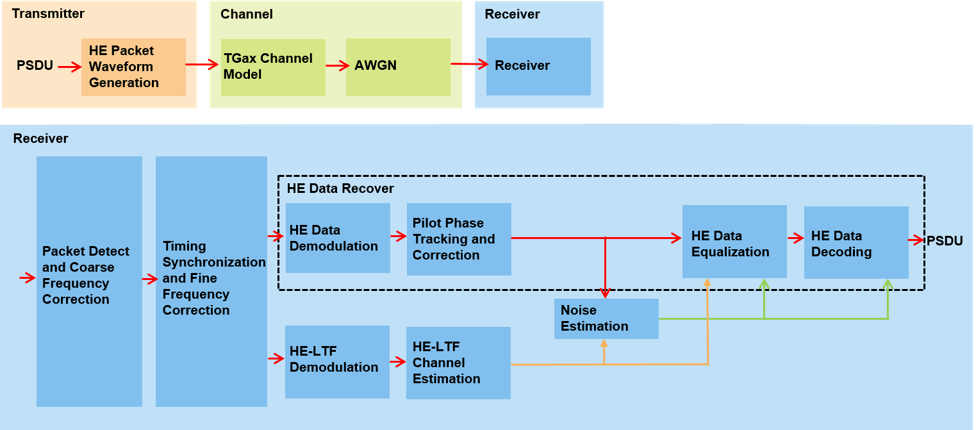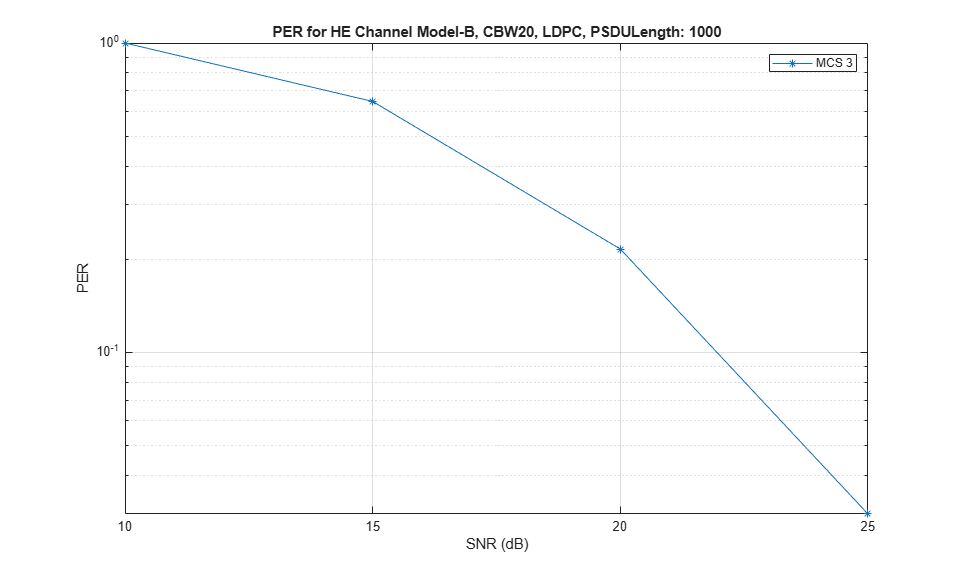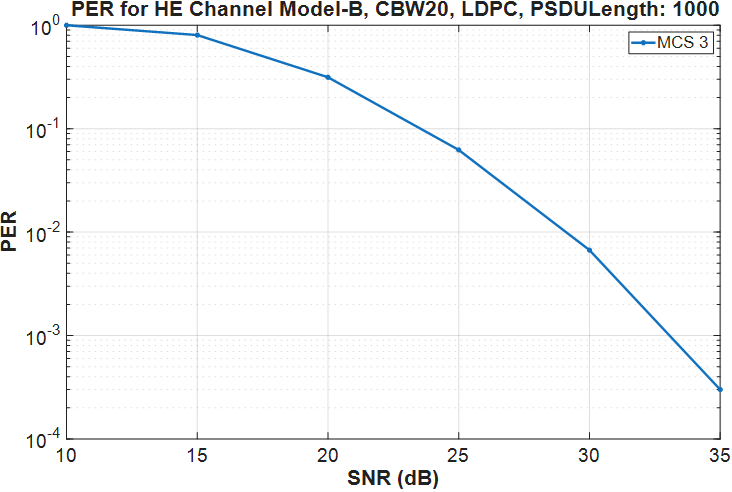## 802.11ax Packet Error Rate Simulation for Single User Format

This example shows how to measure the packet error rate of an IEEE® 802.11ax™ high efficiency (HE) single user format link.

### Introduction

In this example, an end-to-end simulation is used to determine the packet error rate for an 802.11ax [ 1 ] single user format link for a selection of SNR points. At each SNR point, multiple packets are transmitted through a noisy TGax indoor channel, demodulated and the PSDUs recovered. The PSDUs are compared to those transmitted to determine the packet error rate. The processing for each packet is summarized in the following diagram.### Waveform Configuration

An HE single user (SU) packet is a full-band transmission to a single user. The transmit parameters for the HE-SU format are configured using an wlanHESUConfig object. The properties of the object contain the configuration. In this example, the object is configured for a 20 MHz channel bandwidth, 2 transmit antennas, 2 space-time streams, no space time block coding and 16-QAM rate-1/2 (MCS 3).

```cfgHE = wlanHESUConfig; cfgHE.ChannelBandwidth = 'CBW20'; % Channel bandwidth cfgHE.NumSpaceTimeStreams = 2; % Number of space-time streams cfgHE.NumTransmitAntennas = 2; % Number of transmit antennas cfgHE.APEPLength = 1e3; % Payload length in bytes cfgHE.ExtendedRange = false; % Do not use extended range format cfgHE.Upper106ToneRU = false; % Do not use upper 106 tone RU cfgHE.PreHESpatialMapping = false; % Spatial mapping of pre-HE fields cfgHE.GuardInterval = 0.8; % Guard interval duration cfgHE.HELTFType = 4; % HE-LTF compression mode cfgHE.ChannelCoding = 'LDPC'; % Channel coding cfgHE.MCS = 3; % Modulation and coding scheme ```

### Channel Configuration

In this example, a TGax NLOS indoor channel model is used with delay profile Model-B. Model-B is considered NLOS when the distance between transmitter and receiver is greater than or equal to 5 meters. This is described further in wlanTGaxChannel. A 2x2 MIMO channel is simulated in this example.

```% Create and configure the TGax channel chanBW = cfgHE.ChannelBandwidth; tgaxChannel = wlanTGaxChannel; tgaxChannel.DelayProfile = 'Model-B'; tgaxChannel.NumTransmitAntennas = cfgHE.NumTransmitAntennas; tgaxChannel.NumReceiveAntennas = 2; tgaxChannel.TransmitReceiveDistance = 5; % Distance in meters for NLOS tgaxChannel.ChannelBandwidth = chanBW; tgaxChannel.LargeScaleFadingEffect = 'None'; fs = wlanSampleRate(cfgHE); tgaxChannel.SampleRate = fs; ```

### Simulation Parameters

For each SNR point (dB) in the `snr` vector a number of packets are generated, passed through a channel and demodulated to determine the packet error rate.

```snr = 10:5:35; ```

The number of packets tested at each SNR point is controlled by two parameters:

1. `maxNumErrors` is the maximum number of packet errors simulated at each SNR point. When the number of packet errors reaches this limit, the simulation at this SNR point is complete.

2. `maxNumPackets` is the maximum number of packets simulated at each SNR point and limits the length of the simulation if the packet error limit is not reached.

The numbers chosen in this example will lead to a very short simulation. For statistically meaningful results we recommend increasing these numbers.

```maxNumErrors = 10; % The maximum number of packet errors at an SNR point maxNumPackets = 100; % The Maximum number of packets at an SNR point ```

### Processing SNR Points

For each SNR point a number of packets are tested and the packet error rate calculated. The pre-HE preamble of 802.11ax is backwards compatible with 802.11ac™, therefore in this example the front-end synchronization components for a VHT waveform are used to synchronize the HE waveform at the receiver. For each packet the following processing steps occur:

1. A PSDU is created and encoded to create a single packet waveform.

2. The waveform is passed through an indoor TGax channel model. Different channel realizations are modeled for different packets.

3. AWGN is added to the received waveform to create the desired average SNR per subcarrier after OFDM demodulation. comm.AWGNChannel is configured to provide the correct SNR. The configuration accounts for normalization within the channel by the number of receive antennas, and the noise energy in unused subcarriers which are removed during OFDM demodulation.

4. The packet is detected.

5. Coarse carrier frequency offset is estimated and corrected.

6. Fine timing synchronization is established. The L-STF, L-LTF and L-SIG samples are provided for fine timing to allow for packet detection at the start or end of the L-STF.

7. Fine carrier frequency offset is estimated and corrected.

8. The HE-LTF is extracted from the synchronized received waveform. The HE-LTF is OFDM demodulated and channel estimation is performed.

9. The data field is extracted from the synchronized received waveform and OFDM demodulated.

10. Common phase error pilot tracking is performed to track any residual carrier frequency offset.

11. Noise estimation is performed using the demodulated data field pilots and single-stream channel estimate at pilot subcarriers.

12. The phase corrected OFDM symbols are equalized with the channel estimate.

13. The equalized symbols are demodulated and decoded to recover the PSDU.

A parfor loop can be used to parallelize processing of the SNR points, therefore for each SNR point an AWGN channel is created and configured with comm.AWGNChannel. To enable the use of parallel computing for increased speed comment out the 'for' statement and uncomment the 'parfor' statement below.

```numSNR = numel(snr); % Number of SNR points packetErrorRate = zeros(1,numSNR); % Get occupied subcarrier indices and OFDM parameters ofdmInfo = wlanHEOFDMInfo('HE-Data',cfgHE); % Indices to extract fields from the PPDU ind = wlanFieldIndices(cfgHE); %parfor isnr = 1:numSNR % Use 'parfor' to speed up the simulation for isnr = 1:numSNR % Set random substream index per iteration to ensure that each % iteration uses a repeatable set of random numbers stream = RandStream('combRecursive','Seed',99); stream.Substream = isnr; RandStream.setGlobalStream(stream); % Create an instance of the AWGN channel per SNR point simulated awgnChannel = comm.AWGNChannel; awgnChannel.NoiseMethod = 'Signal to noise ratio (SNR)'; awgnChannel.SignalPower = 1/tgaxChannel.NumReceiveAntennas; % Account for noise energy in nulls so the SNR is defined per % active subcarrier awgnChannel.SNR = snr(isnr)-10*log10(ofdmInfo.FFTLength/ofdmInfo.NumTones); % Loop to simulate multiple packets numPacketErrors = 0; numPkt = 1; % Index of packet transmitted while numPacketErrors<=maxNumErrors && numPkt<=maxNumPackets % Generate a packet with random PSDU psduLength = getPSDULength(cfgHE); % PSDU length in bytes txPSDU = randi([0 1],psduLength*8,1); tx = wlanWaveformGenerator(txPSDU,cfgHE); % Add trailing zeros to allow for channel delay txPad = [tx; zeros(50,cfgHE.NumTransmitAntennas)]; % Pass through a fading indoor TGax channel reset(tgaxChannel); % Reset channel for different realization rx = tgaxChannel(txPad); % Pass the waveform through AWGN channel rx = awgnChannel(rx); % Packet detect and determine coarse packet offset coarsePktOffset = wlanPacketDetect(rx,chanBW); if isempty(coarsePktOffset) % If empty no L-STF detected; packet error numPacketErrors = numPacketErrors+1; numPkt = numPkt+1; continue; % Go to next loop iteration end % Extract L-STF and perform coarse frequency offset correction lstf = rx(coarsePktOffset+(ind.LSTF(1):ind.LSTF(2)),:); coarseFreqOff = wlanCoarseCFOEstimate(lstf,chanBW); rx = helperFrequencyOffset(rx,fs,-coarseFreqOff); % Extract the non-HT fields and determine fine packet offset nonhtfields = rx(coarsePktOffset+(ind.LSTF(1):ind.LSIG(2)),:); finePktOffset = wlanSymbolTimingEstimate(nonhtfields,chanBW); % Determine final packet offset pktOffset = coarsePktOffset+finePktOffset; % If packet detected outwith the range of expected delays from % the channel modeling; packet error if pktOffset>50 numPacketErrors = numPacketErrors+1; numPkt = numPkt+1; continue; % Go to next loop iteration end % Extract L-LTF and perform fine frequency offset correction rxLLTF = rx(pktOffset+(ind.LLTF(1):ind.LLTF(2)),:); fineFreqOff = wlanFineCFOEstimate(rxLLTF,chanBW); rx = helperFrequencyOffset(rx,fs,-fineFreqOff); % HE-LTF demodulation and channel estimation rxHELTF = rx(pktOffset+(ind.HELTF(1):ind.HELTF(2)),:); heltfDemod = wlanHEDemodulate(rxHELTF,'HE-LTF',cfgHE); [chanEst,pilotEst] = heLTFChannelEstimate(heltfDemod,cfgHE); % Data demodulate rxData = rx(pktOffset+(ind.HEData(1):ind.HEData(2)),:); demodSym = wlanHEDemodulate(rxData,'HE-Data',cfgHE); % Pilot phase tracking demodSym = heCommonPhaseErrorTracking(demodSym,chanEst,cfgHE); % Estimate noise power in HE fields nVarEst = heNoiseEstimate(demodSym(ofdmInfo.PilotIndices,:,:),pilotEst,cfgHE); % Extract data subcarriers from demodulated symbols and channel % estimate demodDataSym = demodSym(ofdmInfo.DataIndices,:,:); chanEstData = chanEst(ofdmInfo.DataIndices,:,:); % Equalization and STBC combining [eqDataSym,csi] = heEqualizeCombine(demodDataSym,chanEstData,nVarEst,cfgHE); % Recover data rxPSDU = wlanHEDataBitRecover(eqDataSym,nVarEst,csi,cfgHE); % Determine if any bits are in error, i.e. a packet error packetError = ~isequal(txPSDU,rxPSDU); numPacketErrors = numPacketErrors+packetError; numPkt = numPkt+1; end % Calculate packet error rate (PER) at SNR point packetErrorRate(isnr) = numPacketErrors/(numPkt-1); disp(['MCS ' num2str(cfgHE.MCS) ','... ' SNR ' num2str(snr(isnr)) ... ' completed after ' num2str(numPkt-1) ' packets,'... ' PER:' num2str(packetErrorRate(isnr))]); end ```
```MCS 3, SNR 10 completed after 11 packets, PER:1 MCS 3, SNR 15 completed after 17 packets, PER:0.64706 MCS 3, SNR 20 completed after 37 packets, PER:0.2973 MCS 3, SNR 25 completed after 100 packets, PER:0.03 MCS 3, SNR 30 completed after 100 packets, PER:0 MCS 3, SNR 35 completed after 100 packets, PER:0 ```

### Plot Packet Error Rate vs SNR

```figure; semilogy(snr,packetErrorRate,'-*'); hold on; grid on; xlabel('SNR (dB)'); ylabel('PER'); dataStr = arrayfun(@(x)sprintf('MCS %d',x),cfgHE.MCS,'UniformOutput',false); legend(dataStr); title(sprintf('PER for HE Channel %s, %s, %s, PSDULength: %d',tgaxChannel.DelayProfile,cfgHE.ChannelBandwidth,cfgHE.ChannelCoding,cfgHE.APEPLength)); ```The number of packets tested at each SNR point is controlled by two parameters: `maxNumErrors` and `maxNumPackets`. For meaningful results, these values should be larger than those presented in this example. As an example, the figure below was created by running a longer simulation with `maxNumErrors`:1e3 and `maxNumPackets`:1e4.### Appendix

This example uses the following helper functions:

### Selected Bibliography

1. IEEE P802.11ax™/D3.1 Draft Standard for Information technology - Telecommunications and information exchange between systems - Local and metropolitan area networks - Specific requirements - Part 11: Wireless LAN Medium Access Control (MAC) and Physical Layer (PHY) Specifications - Amendment 6: Enhancements for High Efficiency WLAN.

##### Support평가판 신청